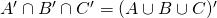# MAE 106 (2018) – Assignment 5

1. Draw the Venn diagram for three sets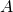,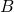, and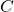that are mutually exclusive.
2. Determine whether the following statements are true or false. Use a Venn diagram to aid you in answering.
• If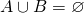, then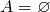, and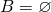.
•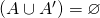• If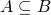, then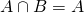.
• Ifand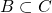, then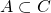.
5. Using the rules of set algebra show the following: